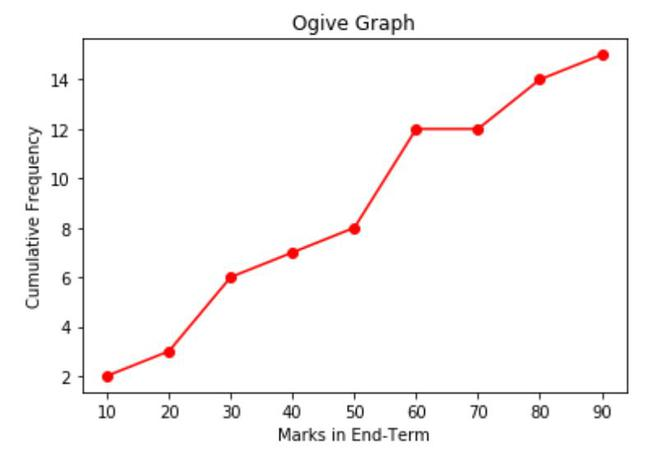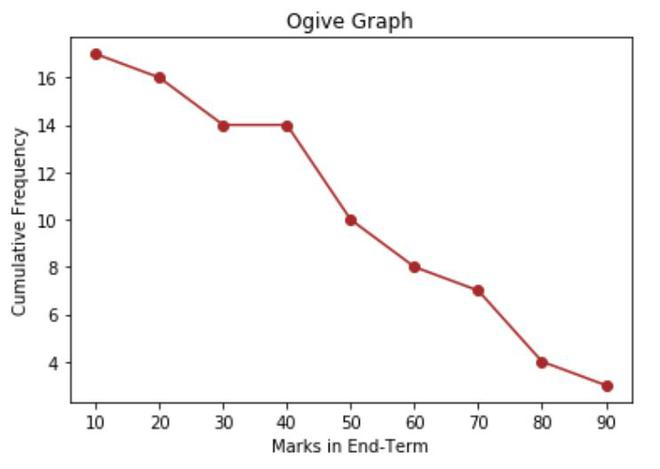# How to Create an Ogive Graph in Python?

• Difficulty Level : Hard
• Last Updated : 08 Mar, 2021

In this article, we will create an Ogive Graph. An ogive graph can also be called as cumulative histograms, this graph is used to determine the number of values that lie above or below a particular value in a data set. The class interval is plotted on the x-axis whereas the cumulative frequency is plotted on the y-axis. These points are plotted on the graph and joined by lines.

NumPy has a function named a histogram() that represents the frequency of data in a particular set range graphically. The histogram function returns two values first one is the frequency and which is stored in values and the second one is the bin values or the interval between which the numbers from the dataset lie, it is stored in the base variable.

After this we will calculate the cumulative sum which can be done easily with the cumsum() function, it returns the cumulative sum along a particular axis. At last, we will plot the graph using plot() function and passing base as x-axis value and cumsum as y-axis value. We can format the graph using markers, color, and linestlye attributes.

Example 1: (More than Ogive graph)

The more than ogive graph shows the number of values greater than the class intervals. The resultant graph shows the number of values in between the class interval. Eg- 0-10,10-20 and so on. Let us take a dataset, and we will now plot it’s more than ogive graph- [22,87,5,43,56,73, 55,54,11,20,51,5,79,31,27].

Table representing intervals, frequency and cumulative frequency(less than)-

Approach:

• Import the modules (matplotlib and numpy).
• Calculate the frequency and cumulative frequency of the data.
• Plot it using the plot() function.

## Python3

 `# importing modules``import` `numpy as np``import` `matplotlib.pyplot as plt`` ` `# creating dataset``data ``=` `[``22``, ``87``, ``5``, ``43``, ``56``, ``73``, ``55``, ``54``, ``11``, ``20``, ``51``, ``5``, ``79``, ``31``, ``27``]`` ` `# creating class interval``classInterval ``=` `[``0``, ``10``, ``20``, ``30``, ``40``, ``50``, ``60``, ``70``, ``80``, ``90``]`` ` `# calculating frequency and class interval``values, base ``=` `np.histogram(data, bins``=``classInterval)`` ` `# calculating cumulative sum``cumsum ``=` `np.cumsum(values)`` ` `# plotting  the ogive graph``plt.plot(base[``1``:], cumsum, color``=``'red'``, marker``=``'o'``, linestyle``=``'-'``)`` ` `# formatting``plt.title(``'Ogive Graph'``)``plt.xlabel(``'Marks in End-Term'``)``plt.ylabel(``'Cumulative Frequency'``)`

Output:Example 2: (Less than Ogive Graph)

In this example, we will plot less than Ogive graph which will show the less than values of class intervals. Dataset:[44,27,5,2,43,56,77,53,89,54,11,23, 51,5,79,25,39]

Table representing intervals, frequency and cumulative frequency(more than)-

Approach is same as above only the cumulative sum that we will calculate will be reversed using flipud() function present in the numpy library.

## Python3

 `# importing modules``import` `numpy as np``import` `matplotlib.pyplot as plt`` ` `# creating dataset``data ``=` `[``44``, ``27``, ``5``, ``2``, ``43``, ``56``, ``77``, ``53``, ``89``, ``54``, ``11``, ``23``, ``51``, ``5``, ``79``, ``25``, ``39``]`` ` `# creating class interval``classInterval ``=` `[``0``, ``10``, ``20``, ``30``, ``40``, ``50``, ``60``, ``70``, ``80``, ``90``]`` ` `# calculating frequency and intervals``values, base ``=` `np.histogram(data, bins``=``classInterval)`` ` `# calculating cumulative frequency``cumsum ``=` `np.cumsum(values)`` ` `# reversing cumulative frequency``res ``=` `np.flipud(cumsum)`` ` `# plotting ogive``plt.plot(base[``1``:], res, color``=``'brown'``, marker``=``'o'``, linestyle``=``'-'``)`` ` `# formatting the graph``plt.title(``'Ogive Graph'``)``plt.xlabel(``'Marks in End-Term'``)``plt.ylabel(``'Cumulative Frequency'``)`

Output:My Personal Notes arrow_drop_up